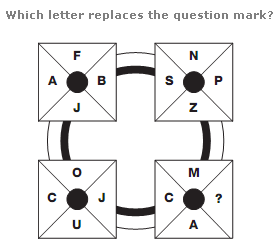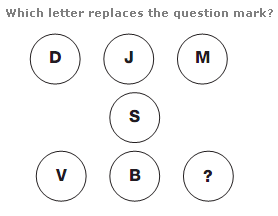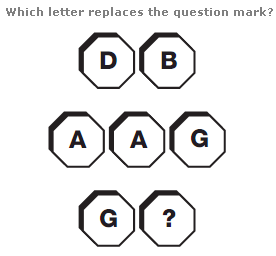# Puzzles - Missing letters puzzles

### Exercise :: Missing letters puzzlesAnswer : F Explanation : Start with the A in the top left hand segment, and move in straight vertical lines, from top to bottom, left to right. Letters follow the alphabetic sequence, skipping 1 letter, then 2, then 3 etc.Answer : E Explanation : Start at the top left, and move around the diagram in a Z shape. The numerical value of the letters increases alternately by 6, then 3.Answer : E Explanation : Convert each letter to its numerical value, and split the diagram into rows, reading each row as a 2 or 3 digit value. The value represented by the middle line of the diagram equals the sum of the upper value and lower value.